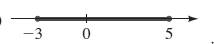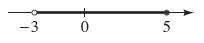# To express: the given graph in interval notation.### Precalculus: Mathematics for Calcu...

6th Edition
Stewart + 5 others
Publisher: Cengage Learning
ISBN: 9780840068071### Precalculus: Mathematics for Calcu...

6th Edition
Stewart + 5 others
Publisher: Cengage Learning
ISBN: 9780840068071

#### Solutions

Chapter 1.1, Problem 57E

a)

To determine

## To express: the given graph in interval notation.

Expert Solution

In terms of interval the setcan be expressed as [3,5] .

### Explanation of Solution

Given information:

Agraphis given asConcept used:

Aninequality a<x<b in terms of an interval can be expressed as (a,b) .

Aninequality axb in terms of an interval can be expressed as [a,b] .

Aninequality ax<b in terms of an interval can be expressed as [a,b) .

Aninequality a<xb in terms of an interval can be expressed as (a,b] .

Aninequality xb in terms of an interval can be expressed as (,b] .

Aninequality x<b in terms of an interval can be expressed as (,b) .

Aninequality xa in terms of an interval can be expressed as [a,) .

Aninequality x>a in terms of an interval can be expressed as (a,) .

If the terminal number or point is included in the interval it can be represented by closed ball on the number line otherwise it will be represented by a open ball.

Calculation:

Consider the given graph.Here, for both 3 and 5closed ball are placed on the number line, so both will be included to the interval.

So, the set can be expressed as aninterval as [3,5] .

b)

To determine

### To express: the given graph in interval notation.

Expert Solution

In terms of interval the set can be expressed as (3,5] .

### Explanation of Solution

Given information:

A graph is given asConcept used:

Aninequality a<x<b in terms of an interval can be expressed as (a,b) .

Aninequality axb in terms of an interval can be expressed as [a,b] .

Aninequality ax<b in terms of an interval can be expressed as [a,b) .

Aninequality a<xb in terms of an interval can be expressed as (a,b] .

Aninequality xb in terms of an interval can be expressed as (,b] .

Aninequality x<b in terms of an interval can be expressed as (,b) .

Aninequality xa in terms of an interval can be expressed as [a,) .

Aninequality x>a in terms of an interval can be expressed as (a,) .

If the terminal number or point is included in the interval it can be represented by closed ball on the number line otherwise it will be represented by a open ball.

Calculation:

Consider the given graph.Here, for 3 open ball is placed on the number line, for 5 closed ball is placed, so5 will be included to the interval and 3 is not included in the set.

So, the set can be expressed as aninterval as (3,5] .

### Have a homework question?

Subscribe to bartleby learn! Ask subject matter experts 30 homework questions each month. Plus, you’ll have access to millions of step-by-step textbook answers!